# DIRECTIONS COSINES AND DIRECTION RATIOS INTERMEDIATE FIRST YEAR MATHS FIRST YEAR 1B PROBLEMS WITH SOLUTIONS

Solutions for intermediate mathematics first year 1A and 1B some chapters. The solutions are very simple to understand.

First year 1A : Functions, mathematical induction, addition of vectors, trigonometric ratios upto transformations, trigonometric equations, inverse trigonometric functions, hyperbolic functions and properties of triangles.

First year 1B : Locus,transformation of axes, three dimensional coordinates, the straight line, the planes, pair of straight lines and applications of derivatives.

Textbook solutions for

Direction Cosines and Direction Ratios

Exercise 6(a)

Exercise 6(b)

You can see the solutions for junior inter maths 1B

1. Locus

The straight line sa

Straight lines la

11. Rate measure

You can also see the solutions for junior inter maths 1A

3. Matrices

You can see the solutions for text book Maths 1A

Functions

Exercise 1(a)

Exercise 1(b)

Exercise 1(c)

Mathematical Induction

Exercise 2(a)

Matrices

Exercise 3(a)

Exercise 3(b)

Exercise 3(c)

Exercise 3(d)

Exercise 3(e)

Exercise 3(f)

Exercise 3(g)

Exercise 3(h)

Exercise 3(i)

Exercise 4(a)

Exercise 4(b)

Product of vectors

Exercise 5(a)

Exercise 5(b)

Exercise 5(c)

Trigonometric Ratios up to Transformations

Exercise 6(a)

Exercise 6(b)

Exercise 6(c)

Exercise 6(d)

Exercise 6(e)

Exercise 6(f)

Trigonometric Equations

Exercise 7(a)

Inverse Trigonometric Equations

Exercise 8(a)

Hyperbolic Functions

Exercise 9(a)

Properties of Triangles

Exercise 10(a)

Exercise 10(b)

Maths 1B solutions for

Locus

Exercise 1(a)

Transformation of Axes

Exercise 2(a)

Three Dimensional coordinates

Exercise 5(a)

Exercise 5(b)

Direction Cosines and Direction Ratios

Exercise 6(a)

Exercise 6(b)

The Plane

Exercise 7(a)

Limits and Continuity

Exercise 8(a)

Exercise 8(b)

Exercise 8(c)

Exercise 8(d)

Exercise 8(e)

M

## DIRECTIONS COSINES AND DIRECTION RATIOS INTERMEDIATE FIRST YEAR MATHS FIRST YEAR 1B PROBLEMS WITH SOLUTIONS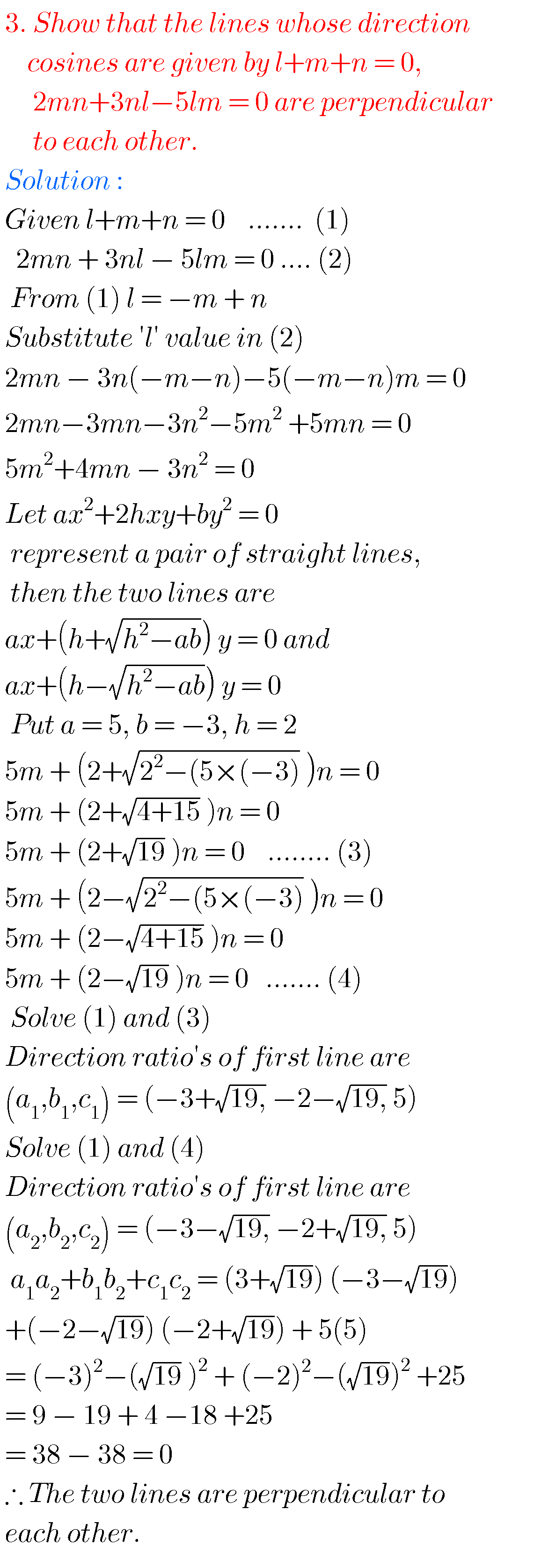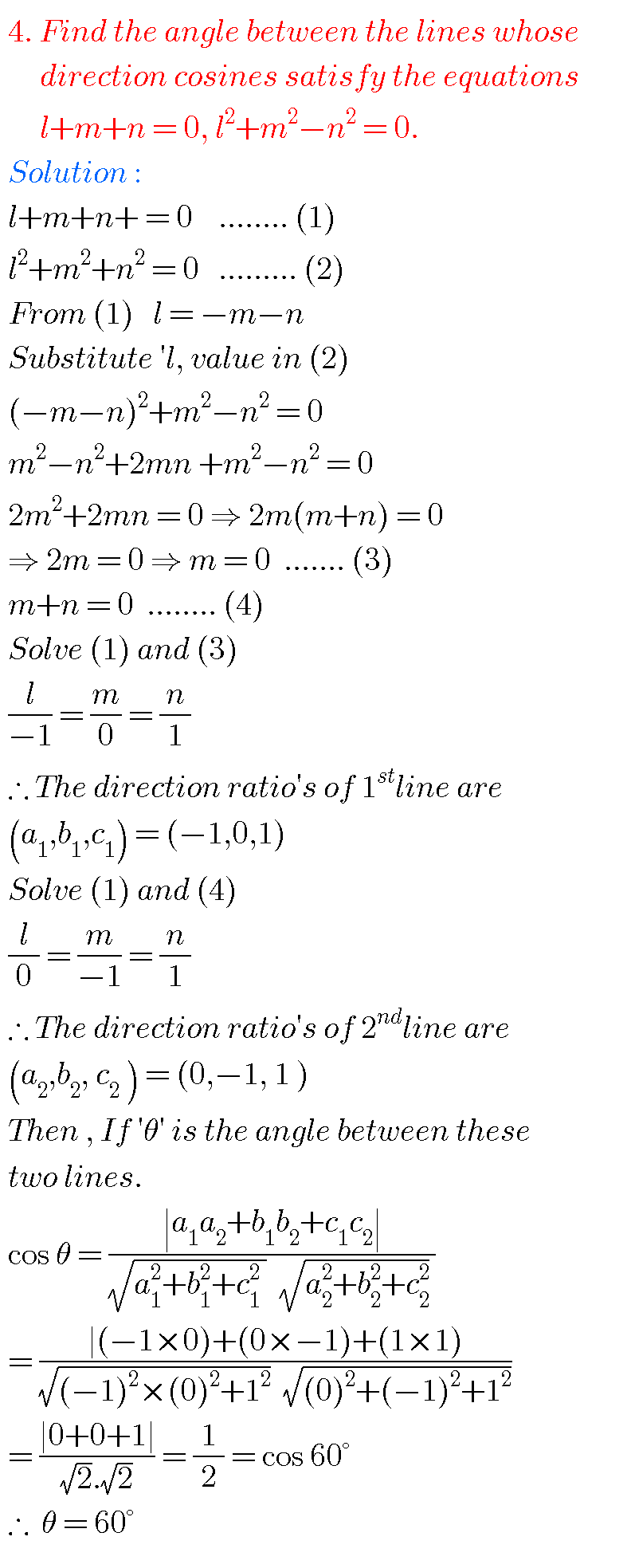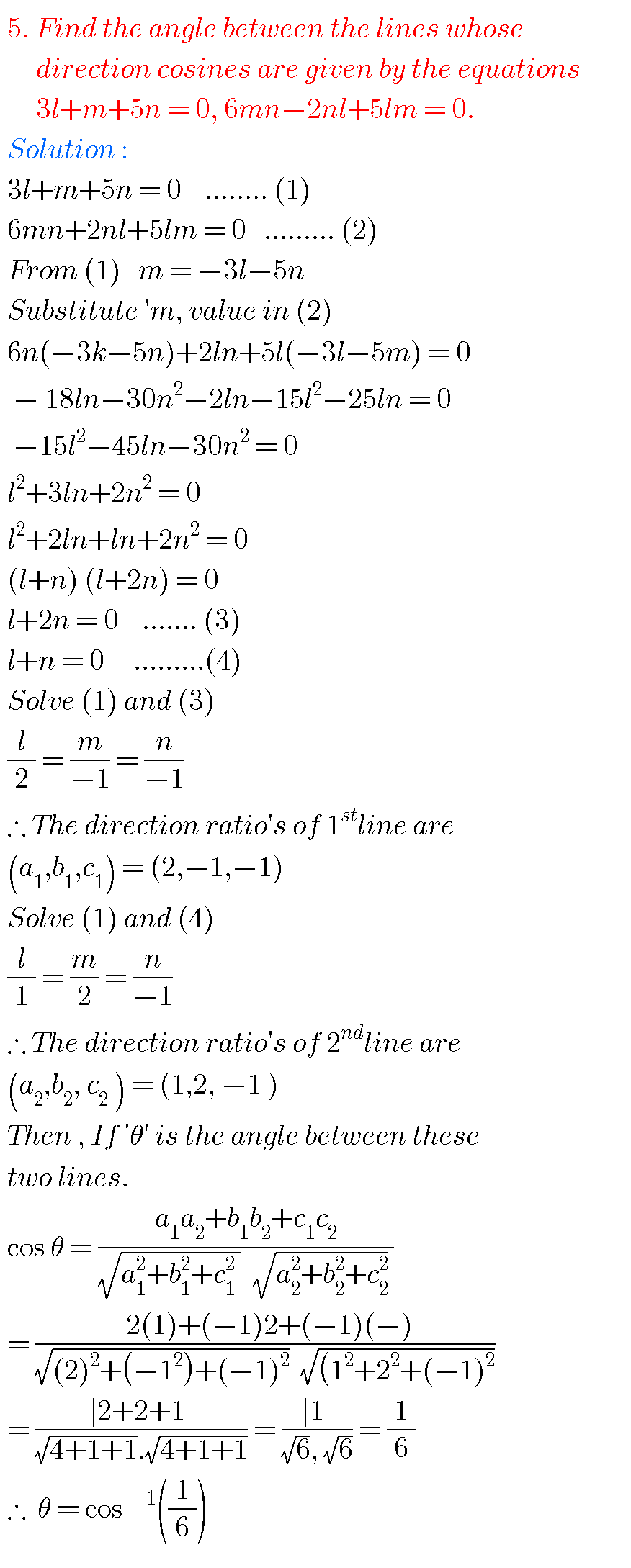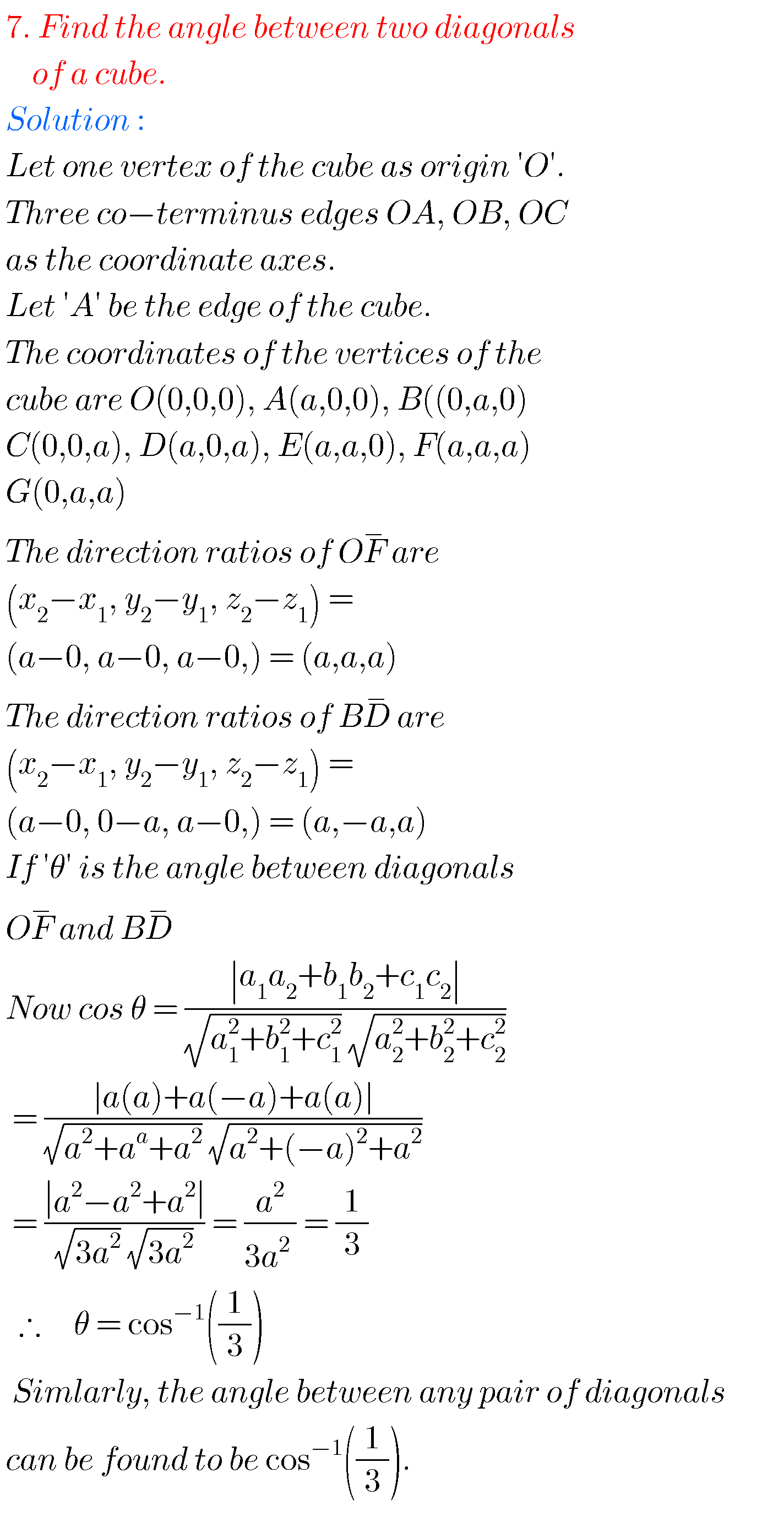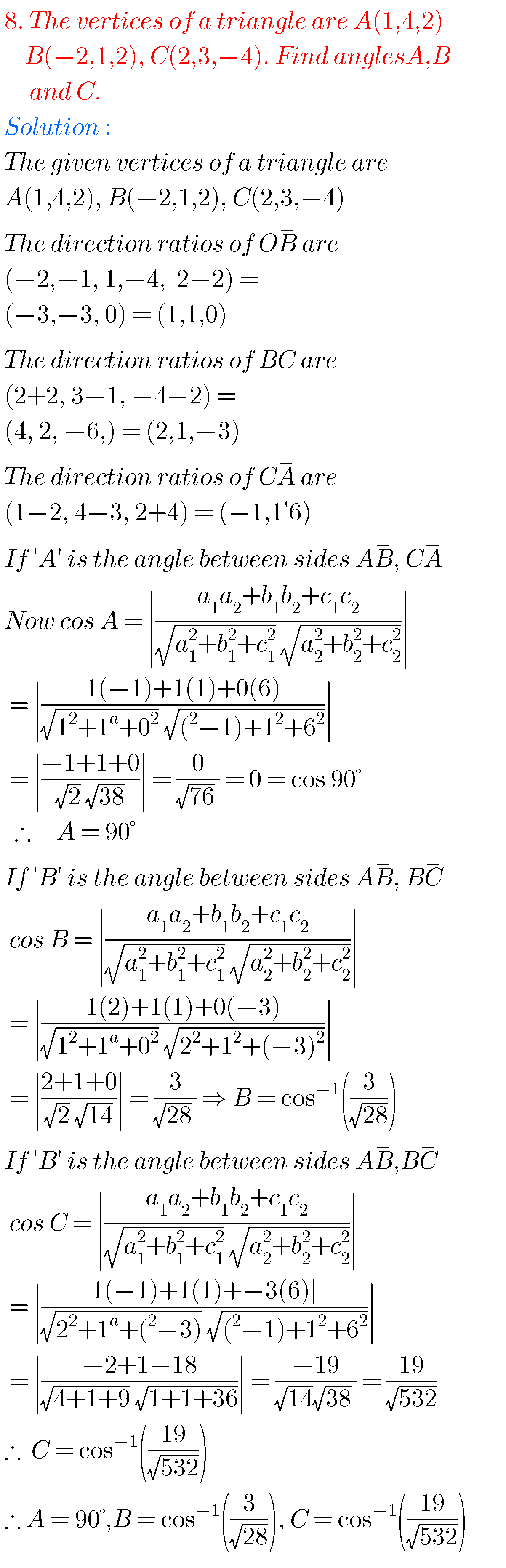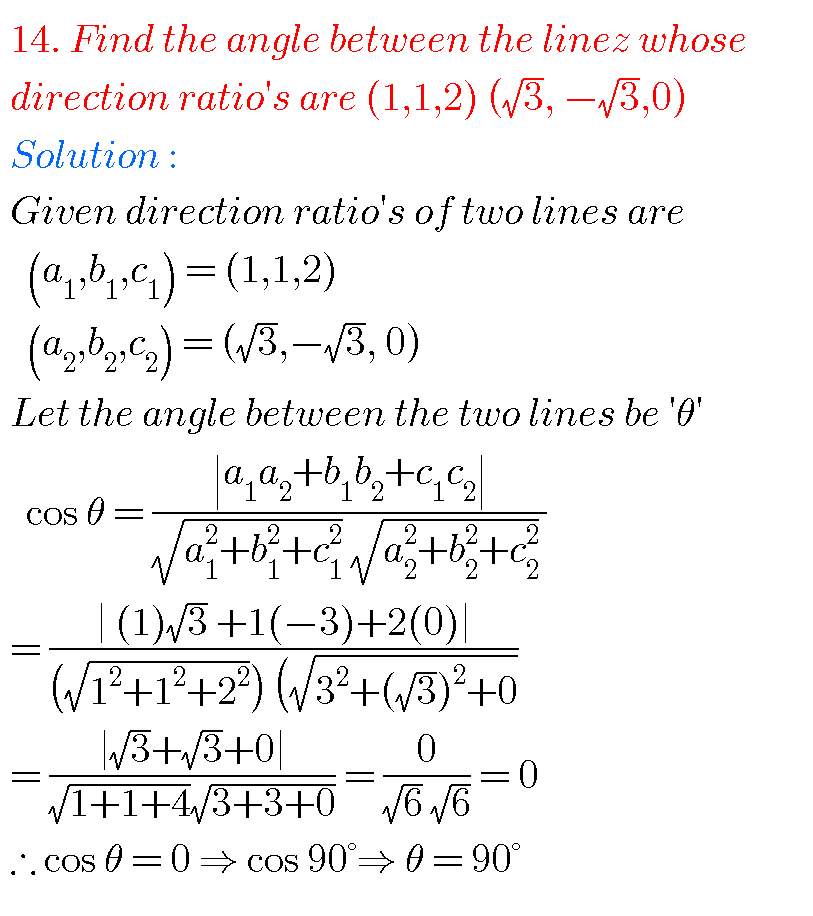Note : Observe the solutions and try them in your own methods.

Inter maths 1a solutions

Inter maths trigonometry solutions

Maths real numbers solutions class 10

You can see solutions for Inter Maths IIB

1. Circle

3. Parabola

4. Ellipse

You can also see solutions for Inter Maths IIA

For examination purpose you can see

Complex numbers

De Moivre’ s Theorem